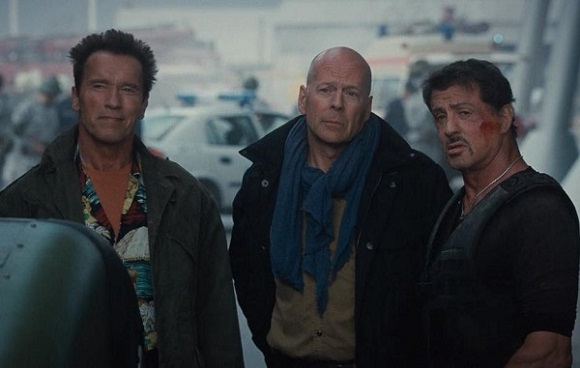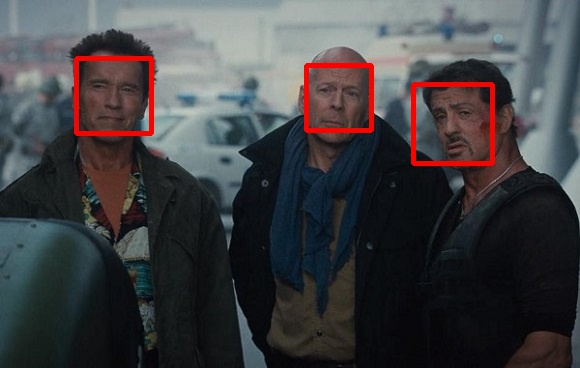# OpenCV - Face Detection in a Picture

The VideoCapture class of the org.opencv.videoio package contains classes and methods to capture video using the system camera. Let’s go step by step and learn how to do it.

## Step 1: Load the OpenCV native library

While writing Java code using OpenCV library, the first step you need to do is to load the native library of OpenCV using the loadLibrary(). Load the OpenCV native library as shown below.

```// Loading the core library
```

## Step 2: Instantiate the CascadeClassifier class

The CascadeClassifier class of the package org.opencv.objdetect is used to load the classifier file. Instantiate this class by passing the xml file lbpcascade_frontalface.xml as shown below.

```// Instantiating the CascadeClassifier
```

## Step 3: Detect the faces

You can detect the faces in the image using method detectMultiScale() of the class named CascadeClassifier. This method accepts an object of the class Mat holding the input image and an object of the class MatOfRect to store the detected faces.

```// Detecting the face in the snap
MatOfRect faceDetections = new MatOfRect();
classifier.detectMultiScale(src, faceDetections);
```

### Example

The following program demonstrates how to detect faces in an image.

```import org.opencv.core.Core;
import org.opencv.core.Mat;
import org.opencv.core.MatOfRect;
import org.opencv.core.Point;
import org.opencv.core.Rect;
import org.opencv.core.Scalar;

import org.opencv.imgcodecs.Imgcodecs;
import org.opencv.imgproc.Imgproc;

public class FaceDetectionImage {
public static void main (String[] args) {

// Reading the Image from the file and storing it in to a Matrix object
String file ="E:/OpenCV/chap23/facedetection_input.jpg";

// Detecting the face in the snap
MatOfRect faceDetections = new MatOfRect();
classifier.detectMultiScale(src, faceDetections);
System.out.println(String.format("Detected %s faces",
faceDetections.toArray().length));

// Drawing boxes
for (Rect rect : faceDetections.toArray()) {
Imgproc.rectangle(
src,                                               // where to draw the box
new Point(rect.x, rect.y),                            // bottom left
new Point(rect.x + rect.width, rect.y + rect.height), // top right
new Scalar(0, 0, 255),
3                                                     // RGB colour
);
}

// Writing the image
Imgcodecs.imwrite("E:/OpenCV/chap23/facedetect_output1.jpg", src);

System.out.println("Image Processed");
}
}
```

Assume that following is the input image facedetection_input.jpg specified in the above program.### Output

On executing the program, you will get the following output −

```Detected 3 faces
Image Processed
```

If you open the specified path, you can observe the output image as follows −